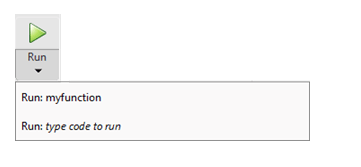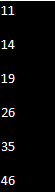GFG App
Open AppBrowser
Continue

# Configure the Run Button for Functions in MATLAB

Functions are parts of program files that process inputs and generate outputs. Set the Run button to execute any additional Editor setup or functions that need argument values to be entered. To configure the Editor’s Run button, click Run and type one or more run commands. We’ll examine a setup example for the function’s run button.

## Steps for Configure the Run Button

Make the function myfunction.m, which uses the inputs a and b to calculate and store the results in c.

## Matlab

 `% MATLAB code for Configure the Run ` `% Button for Functions ` `function` `c=myfunction(a,b) ` `c=a.^2 +b;`

Output:

After selecting the Editor tab, select Run. MATLAB displays the list of commands that can be used to execute the function.Explanation:

• A call to the function with the necessary input arguments should be substituted for the text-typed code to run by clicking the last item on the list.
• To execute all of the commands at once, enter multiple commands on the same line. For instance, enter x = 1:7; y = 10; Use the expression result = myfunction(x,y) to create the variables x and y, and then call myfunction with x and y as the input arguments to create them.
• Choose the option to run. MATLAB uses the first run command in the list to execute the function. For instance, click Run to carry out the command result = myfunction(1:7,10). MATLAB displays the outcome in the Command Window.
• To run the function with a different run command from the list, click Run and select the desired run command. The default run command for the Run button is the one you choose from the list.
• Select Edit or Delete from the context menu by right-clicking on an existing run command, then click Run.

Note: The run command variable takes the place of the base workspace variable when a run command that you define creates a variable with the same name is executed.

My Personal Notes arrow_drop_up